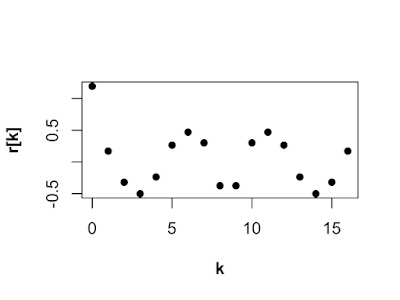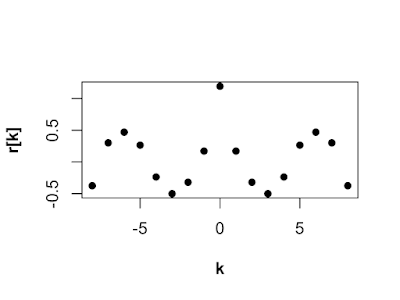## Friday, May 24, 2019

### Random Autocorrelation Sequences R version

Random Autocorrelation Sequences R version

### What is an autocorrelation sequence?

Autocorrelation sequences (ACSs) are super common when doing anything in probability and statistics. Autocorrelation is a sequence of measurements of how similar a sequence is to it self. In math the autocorrelation sequence r[k] is

r[k] = E[x[n]x[n+k]] for k={0,1,...N-1},

where N is the number of data samples, E is the expected value, x[n] is a data sample and k is the lag. The lag is the separation in samples.

### Why make a random autocorrelation sequence?

When testing an algorithm or conducting simulations it is often useful to use a random ACS. Generating random a random ACS can be difficult because they have a lot of special properties and if you select a sequence at random, the chance it is a valid ACS is small.

### Trick to making a random autocorrelation sequence

We can use the following property of ACSs to make generating random ACSs easy. The ACS and the power spectral density (PSD) are Fourier transform (FT) pairs. For our purpose here, a PSD is just a function that is positive everywhere. "FT pair" means the FT of an ACS is a PSD and the inverse FT of a PSD is an ACS.

So we can generate a random ACS using the following steps. First, generate a random sequence. Second, square each element, so the sequence is positive. Finally, find the inverse FT of the squared sequence.

### The R code that produces a random ACS

The ACS could be any size, but in this case we want a 9 element sequence.

N <- 9
PSD <- rnorm(N)^2
ACS <- fft(PSD,inverse = TRUE)

The line below outputs the ACS and as you can see it is a complex sequence.

ACS

 0.6183715+0.0000000i -0.1375219+0.1960568i -0.1672163-0.2084656i 0.2199730-0.0977208i
 -0.0281983+0.2615475i -0.0281983-0.2615475i 0.2199730+0.0977208i -0.1672163+0.2084656i
 -0.1375219-0.1960568i

### What if I want a real ACS

If you want a real ACS then the PSD has to be even. So, let's make the sequence even!

PSDeven <- c(PSD,PSD[N:2])
PSDeven

 0.39244438 0.03372487 0.69827518 2.54492084 0.10857537 0.67316837 0.23758708 0.54512337
 0.33152416 0.33152416 0.54512337 0.23758708 0.67316837 0.10857537 2.54492084 0.69827518
 0.03372487

Notice the ACS is still complex. Numerical error causes some imaginary dust we need to clean up.

ACS <- fft(PSDeven,inverse = TRUE)/N
ACS

 1.1931381+0i 0.1714080+0i -0.3200109+0i -0.5007558+0i -0.2372697+0i 0.2647028+0i
 0.4700409+0i 0.3009945+0i -0.3750372+0i -0.3750372+0i 0.3009945+0i 0.4700409+0i
 0.2647028+0i -0.2372697+0i -0.5007558+0i -0.3200109+0i 0.1714080+0i

Clean up the small imaginary part with Re() and now we are ready to plot.

ACS <- Re(ACS)

### Plot of ACS

The figure below is a plot of the ACS from lag k = 0 to 16. In textbooks the ACS would have been plotted from k=-8 to 8, with r in the center.This is the plot of the ACS in the textbook style. Notice, the lag at 0 r is positive and larger than the other lags, a standard property of ACSs. All is well!